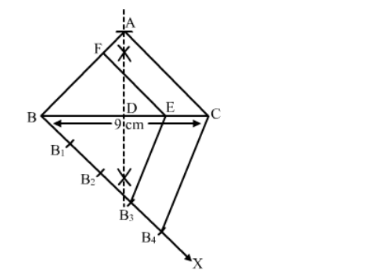# Construct an isosceles triangle whose base is 9 cm and altitude 5 cm.`
Question:

Construct an isosceles triangle whose base is $9 \mathrm{~cm}$ and altitude $5 \mathrm{~cm} .$ Construct another triangle whose sides are $\frac{3}{4}$ the corresponding sides of the first isosceles triangle.

Solution:

Steps of Construction :

Step 1. Draw a line segment BC = 9 cm.
Step 2. With B as centre, draw an arc each above and below BC.
Step 3. With C as centre, draw an arc each above and below BC.
Step 4. Join their points of intersection to obtain the perpendicular bisector of BC. Let it intersect BC at D.
Step 5. From D, cut an arc of radius 5 cm and mark the point as A.
Step 6. Join AB and AC.
Thus, △ABC is obtained .

Step 5 . Below $B C$, make an acute $\angle C B X$.

Step 6. Along BX, mark off four points B1, B2, B3, B4, such that BB1=B1B2 = B2B3 = B3B4.
Step 7. Join B4C.
Step 8. From B3, draw B3E ∥ B4C, meeting BC at E.
Step 9. From E, draw EF ∥ CA, meeting AB at F.Thus, $\triangle F B E$ is the required triangle, each of whose sides is $\frac{3}{4}$ the corresponding sides of the first triangle.